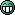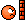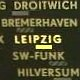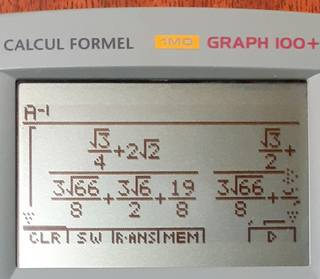# [Question] Looking for the best Casio Calculator for inverse matrices

surds calculator question

5 replies to this topic

### #1blackburnfjames

blackburnfjames

Newbie

•• Members
•• 2 posts

• Calculators:
- fx-86GT PLUS (LY720X VerG)
- fx-991EX CLASSWIZ

Posted 09 October 2021 - 11:55 AM

Hey there!

I hope you are all doing well! (I hope I am posting this in the correct section of the forum)

I am currently moving up to A-level further maths, so I have just moved from an "fx-85GT PLUS" to an "fx-991EX Classwiz", because this is what my school recommends..

The problem is, this CASIO has a serious flaw.

Let us take the following matrix:Now I want to inverse this matrix (which is worth 1 mark in my text book), so I type it into my calculator:As one can see, the calculator has lost any knowledge of surds, and has just done the Decimal calculation. Obviously, for 1 mark, doing a full 3x3 matrix inversion in surd form, is not worth it.

So I started to look for a calculator that could do this (I am happy to buy used, I'm in the UK), and so tried emulating other calculators.

The ones that couldn't do it (from my test, off the top of my head):

- FX-9860G

- Ti-83 (This might be sacrilegious to post on here, but it didn't work, so i might as well)

- FX-9750GIII

So I ended up trying, and I realized that the "fx-9860GIII" could do it!As you can see, it keeps surd form up to this point!But then boom, you inverse it, and it loses all the surd information.

I wonder if any casio calculator can actually do this properly? Or if I need to look at different companies' calculators?

So my question is, is there maybe an older casio model that can do 3x3 matrix inversions whilst keeping surd form?  Or a different company all together?

Or maybe there is a function on the fx-991EX which can do this for us?

I also do love the idea of having a graphing calculator functions, and am a programmer, so I would be interested if these features were available too.

Stay safe and I hope you are all well!

~ James

### #2piu58

piu58

Casio Freak

•• Members
•• 142 posts
• Gender:Male

• Calculators:
Casio fx-9860G, Casio fx-CG20

Posted 09 October 2021 - 05:51 PM

At the cg20 and cg50 calculators you may install xcas, that is symbolic mathematics. Theres is a version for pc and even an online version of the program. I calculated your example with the windows version. I am sure, the calculatore gives the same result:

[

[(-23994*sqrt(2)*sqrt(11)+8832*sqrt(3)*sqrt(11)+68008*sqrt(2)+15326*sqrt(3))/169891,
(-42948*sqrt(2)*sqrt(11)-2544*sqrt(3)*sqrt(11)+179848*sqrt(2)+100844*sqrt(3))/849455,
(-24624*sqrt(2)*sqrt(3)*sqrt(11)+62529*sqrt(2)*sqrt(3)+34074*sqrt(11)+19656)/849455],

[(-20216*sqrt(2)*sqrt(11)-9552*sqrt(3)*sqrt(11)+111112*sqrt(2)-70128*sqrt(3))/169891,
(-60656*sqrt(2)*sqrt(11)-41568*sqrt(3)*sqrt(11)+374256*sqrt(2)-83212*sqrt(3))/849455,
(-17688*sqrt(2)*sqrt(3)*sqrt(11)+92108*sqrt(2)*sqrt(3)-20232*sqrt(11)+192952)/849455],

[(-27576*sqrt(2)*sqrt(11)+3778*sqrt(3)*sqrt(11)+41710*sqrt(2)+43104*sqrt(3))/169891,
(-58536*sqrt(2)*sqrt(11)-17708*sqrt(3)*sqrt(11)-276084*sqrt(2)+194408*sqrt(3))/849455,
(-27153*sqrt(2)*sqrt(3)*sqrt(11)+86648*sqrt(2)*sqrt(3)+20808*sqrt(11)+88737)/849455]

]

Edited by piu58, 09 October 2021 - 05:53 PM.

• blackburnfjames likes this

### #3blackburnfjames

blackburnfjames

Newbie

•• Members
•• 2 posts

• Calculators:
- fx-86GT PLUS (LY720X VerG)
- fx-991EX CLASSWIZ

Posted 11 October 2021 - 07:18 PM

At the cg20 and cg50 calculators you may install xcas, that is symbolic mathematics. Theres is a version for pc and even an online version of the program. I calculated your example with the windows version. I am sure, the calculatore gives the same result:

[

[(-23994*sqrt(2)*sqrt(11)+8832*sqrt(3)*sqrt(11)+68008*sqrt(2)+15326*sqrt(3))/169891,
(-42948*sqrt(2)*sqrt(11)-2544*sqrt(3)*sqrt(11)+179848*sqrt(2)+100844*sqrt(3))/849455,
(-24624*sqrt(2)*sqrt(3)*sqrt(11)+62529*sqrt(2)*sqrt(3)+34074*sqrt(11)+19656)/849455],

[(-20216*sqrt(2)*sqrt(11)-9552*sqrt(3)*sqrt(11)+111112*sqrt(2)-70128*sqrt(3))/169891,
(-60656*sqrt(2)*sqrt(11)-41568*sqrt(3)*sqrt(11)+374256*sqrt(2)-83212*sqrt(3))/849455,
(-17688*sqrt(2)*sqrt(3)*sqrt(11)+92108*sqrt(2)*sqrt(3)-20232*sqrt(11)+192952)/849455],

[(-27576*sqrt(2)*sqrt(11)+3778*sqrt(3)*sqrt(11)+41710*sqrt(2)+43104*sqrt(3))/169891,
(-58536*sqrt(2)*sqrt(11)-17708*sqrt(3)*sqrt(11)-276084*sqrt(2)+194408*sqrt(3))/849455,
(-27153*sqrt(2)*sqrt(3)*sqrt(11)+86648*sqrt(2)*sqrt(3)+20808*sqrt(11)+88737)/849455]

]

That's very interesting, thanks very much!!! I might look into getting one of these calculators!

Another question along the same vein, is, on the fx-991EX, i realized that surds are supported in a matrix!

I.e. when you square a matrix with surds, some whole numbers can be seen. So clearly the calculation is done using surds! My problem is that, numbers are outputted in a matrix as DecimalO. Is there some way to access a specific cell of a matrix ?

I.e.:This suggests that, within the calculator's logic, it keeps the surd logic. My problem is, how can I convert these DecimalO values to MathO.

So I was wondering, is there a way to access each individual value, maybe like such?I tried using STO, but that just stores the whole Matrix, not the individual variables.

Thanks very much for your suggestion of the CG50! I'll see if i can get one at an affordable price!

### #4HappyCalc

HappyCalc

•• Members
•• 57 posts
• Gender:Male
• Location:Germany
• Interests:Programming, On-Calc Programming(C.Basic), Gaming, Origami, Eating Chocolate

• Calculators:
fx-9860GII-2
fx-CG50

Posted 12 October 2021 - 04:27 PM

There is a 9860giii version of xcas(khicas). On my cg50 it works, but i don't have a 9860giii and for the gii the file is too big (~2MB)
https://www-fourier....nstall_en#casio
As piu said, xcas is using symbolic math while the math menu just saves data in 12-byte? (correct me if wrong) BCD-floats. Thus it calculates the decimal and saves it.
• blackburnfjames likes this

### #5Hlib2

Hlib2

Casio Freak

•• Members
•• 139 posts
• Gender:Male
• Location:Ukraine
• Interests:industrial electronics,
graphing calculators

• Calculators:
fx-9860GII-2,
cfx-9850GC+,
fx-9750G+, graph_100+,
fx-9750GII, fx-991DE_X,
ti-83+_SE, ti-84+, ti-85,
ti-89_Titanium,
ti-voyage200.

Posted 13 October 2021 - 07:21 PM

AFAIK, your example can only be solved by a calculator with CAS, at least, afx-2.0/graph100+ or ti-89/v200.• Hlib2 likes this

### #6HappyCalc

HappyCalc

•• Members
•• 57 posts
• Gender:Male
• Location:Germany
• Interests:Programming, On-Calc Programming(C.Basic), Gaming, Origami, Eating Chocolate

• Calculators:
fx-9860GII-2
fx-CG50

Posted 31 October 2021 - 08:11 AM

So I was wondering, is there a way to access each individual value

I don't know if it works on your calculator, but on calculators like 9860g ang cg you can do Mat A[1,1] for accessing top left cell (a bit like an array)

### Also tagged with one or more of these keywords: surds, calculator, question

#### 0 user(s) are reading this topic

0 members, 0 guests, 0 anonymous users